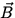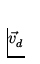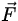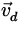# Magnetic Force on a Current Carrying Wire

Let us consider a long straight wire carrying a currentin a magnetic field. Each charge q in the wire will experience a force, and it is possible to find the total force on the wire by the following arguments. Suppose there are n charges per unit volume in the wire of cross-sectional area A and length l , as in Fig. 1.4.If each charge has a charge q and is moving with a drift velocity, then there will be a total force on the wire given by= (nAlq)x= lx, (4)

where we recall that= nqA and also that by conventionis in the direction of the flow of positive charges.